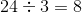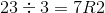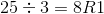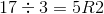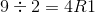SSAT Elementary Level Math : How to divide

Example Questions

Example Question #31 : How To Divide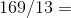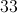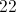Explanation:

Multiply each answer choice by 13 to determine if the product results in 169: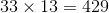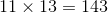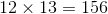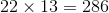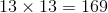Therefore: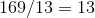Example Question #32 : How To Divide

Nikki has 164 coins in her coin collection. She decides to break her coin collection into 4 equal groups. How many coins are in each group?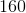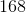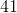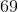Explanation:

Since Nikki is breaking her total number of coins into smaller, equal groups, we need to divide.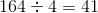Example Question #33 : How To Divide

Trinity decides to break up her coin collection intoequal groups. Her coin collection consists of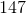coins. How many coins will Trinity put in each group?Explanation:

Because Trinity is breaking her total number of coins into smaller, equal groups, we need to divide!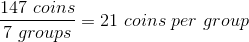Example Question #34 : How To Divide

Jayme hastoy ponies. If she breaks them up intoequal groups, how many toy ponies will be in each group?Explanation:

Because Jayme is breaking her total number of toy ponies into smaller, equal groups, we need to divide!toy ponies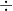groups =toy ponies per group

Example Question #35 : How To Divide

Find the quotient, and show a remainder if necessary. (Remainders are denoted with "R" in the answer choices.)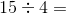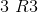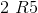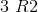Explanation:

When you divide 4 into 15, it does not go in evenly.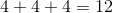4 goes into 15 three full times. The remainder is the difference between 15 and 12.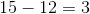Therefore the remainder is 3, so the correct answer is 3 R3.

Example Question #36 : How To Divide

Find the quotient and show a remainder if necessary. (Remainders are denoted with R in the answer choices.)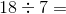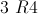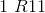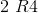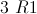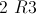Explanation:

7 does not divide evenly into 18, so there will be a remainder. 7 goes into 18 two times.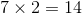To find the remainder, we subtract 14 from 18.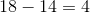The answer is therefore 2 R4.

You can also draw a picture to help visualize the problem:

ooooooo   ooooooo   oooo

This shows 2 groups of 7 (for a total of 14), with 4 left over (the remainder).

Example Question #37 : How To Divide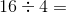Explanation:

Divide 16 by 4. The quotient is 4.

To visualize, divide 16 objects into equal groups of 4, then count how many groups there are:

oooo oooo oooo oooo  = 4 groups in all.

Example Question #38 : How To Divide

What is the result of dividing six by two?

-3

6

3

2

12

3

Explanation:

We want to know how many 2's are in 6.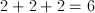Example Question #39 : How To Divide

Bryce hasbaseball cards. He wants to break them up intoequal groups. After breaking his cards up, how many baseball cards will be in each group?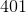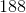Explanation:

Because Bryce is breaking his total number of baseball cards into smaller, equal groups, we need to divide!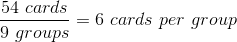Example Question #40 : How To Divide

Which of the following equations does NOT have a remainder?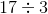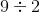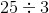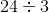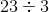Explanation:

A division equation will not have a remainder if a number can be divided evenly by another number.

The only given equation in which there is no remainder isbecause 3 goes into 24 exactly 8 times.